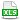HomeTechniques and Tips@RISK DistributionsEntering Parameters for Log-normal Distribution

# 3.29. Entering Parameters for Log-normal Distribution

Applies to:
@RISK, all releases
RISKOPTIMIZER, all releases
RDK and RODK, all releases

The RiskLognorm( ) function doesn't behave like the log-normal function in the books. When I enter μ=4, σ=2, I expect the simulated distribution to have a mean of 54.6 and standard deviation of 2953.5, but instead the mean and standard deviation are very close to 4 and 2. What's wrong?

@RISK, RISKOptimizer, and the developer kits have two log-normal distributions. RiskLognorm2( ) is the traditional distribution and behaves in the way described in statistics books. We also offer RiskLognorm( ), where the μ and σ (mu and sigma) you enter are the actual mean and standard deviation of the distribution, subject to the usual sampling fluctuation. The two distributions are the same except for the way you enter parameters.

• If you know the desired actual mean and standard deviation, use RiskLognorm( ).  For RiskLognorm(μ,σ):
Actual mean of the distribution = μ
Actual standard deviation of the distribution = σ

• If you want to use parameters that match the log-normal distribution in many textbooks, use RiskLognorm2( ).  For RiskLognorm2(μ,σ):
Actual mean of the distribution = exp(μ+σ²/2)
Actual standard deviation of the distribution = exp(μ+σ²/2)·sqrt(exp(σ²)−1) = (actual mean)·sqrt[exp(σ²)−1]

• Finally, if you know the desired geometric mean and standard deviation of a log-normal distribution, use RiskLognorm2( ) but set μ to the natural log of the desired geometric mean, and σ to the natural log of the desired geometric standard deviation.  For details, please see Geometric Mean and Geometric SD in Log-normal.

These three methods are illustrated in the accompanying workbook.

Additional keywords: Lognorm distribution, Lognorm2 distribution

Last edited: 2015-06-19

•LogNormal.xlsx